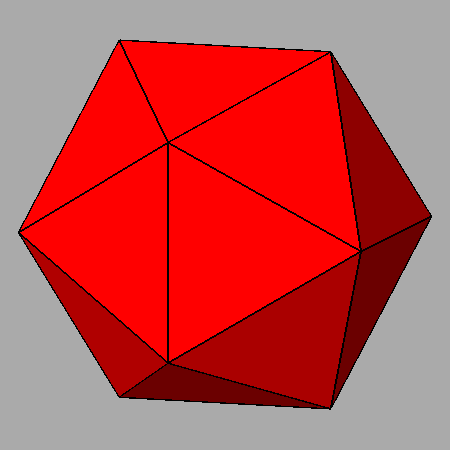Acronym ... Name dodecahedrically truncated rhombic triacontahedron,chamfered icosahedron©© Vertex figure [3,H2], [h5] General of army (is itself convex) Colonel of regiment (is itself locally convex) Confer rhote   ike ExternallinksThe non-regular hexahedra {(h,H,H)2} have vertex angles h = arccos(1/sqrt(5)) = 63.434949° resp. H = arccos(-sqrt[(5+sqrt(5))/10]) = 148.282526°.

Dodecahedral truncation applies to the dodecahedral vertices (vertex inscribed doe) only. Wrt. the rhote this produces new triangle faces there. These are then face planes of a cutting ike. – The above transition shows a dynamical mutual scaling of ike and rhote. The chamfered ike then is the instance, where all edges happen to have the same length.

Chamfering (or edge-only beveling – here being applied to the ike) flatens the former edges into new (non-regular hexagonal) faces.

There is a deeper, terminal chamfering of the ike too, which then reduces the original faces to nothing. Then the hexagons will become rhombs and the total figure becomes the rhote. – When considering the below provided tegum sum Dynkin symbol, it becomes obvious that this figure also can be seen as a Stott expansion of the rhote.

Incidence matrix according to Dynkin symbol

ax3oo5oc&#zx   → height = 0
a = 1+sqrt[(10+2 sqrt(5))/5] = 2.701302
c = sqrt[(10-2 sqrt(5))/5] = 1.051462
(tegum sum of a-ike and (x,c)-srid)

o.3o.5o.     | 12  * |  5  0 |  5  0  [h5]
.o3.o5.o     |  * 60 |  1  2 |  2  1  [3,H2]
-------------+-------+-------+------
oo3oo5oo&#x  |  1  1 | 60  * |  2  0
.x .. ..     |  0  2 |  * 60 |  1  1
-------------+-------+-------+------
ax .. oc&#zx |  2  4 |  4  2 | 30  *  {(h,H,H)2}
.x3.o ..     |  0  3 |  0  3 |  * 20  {3}